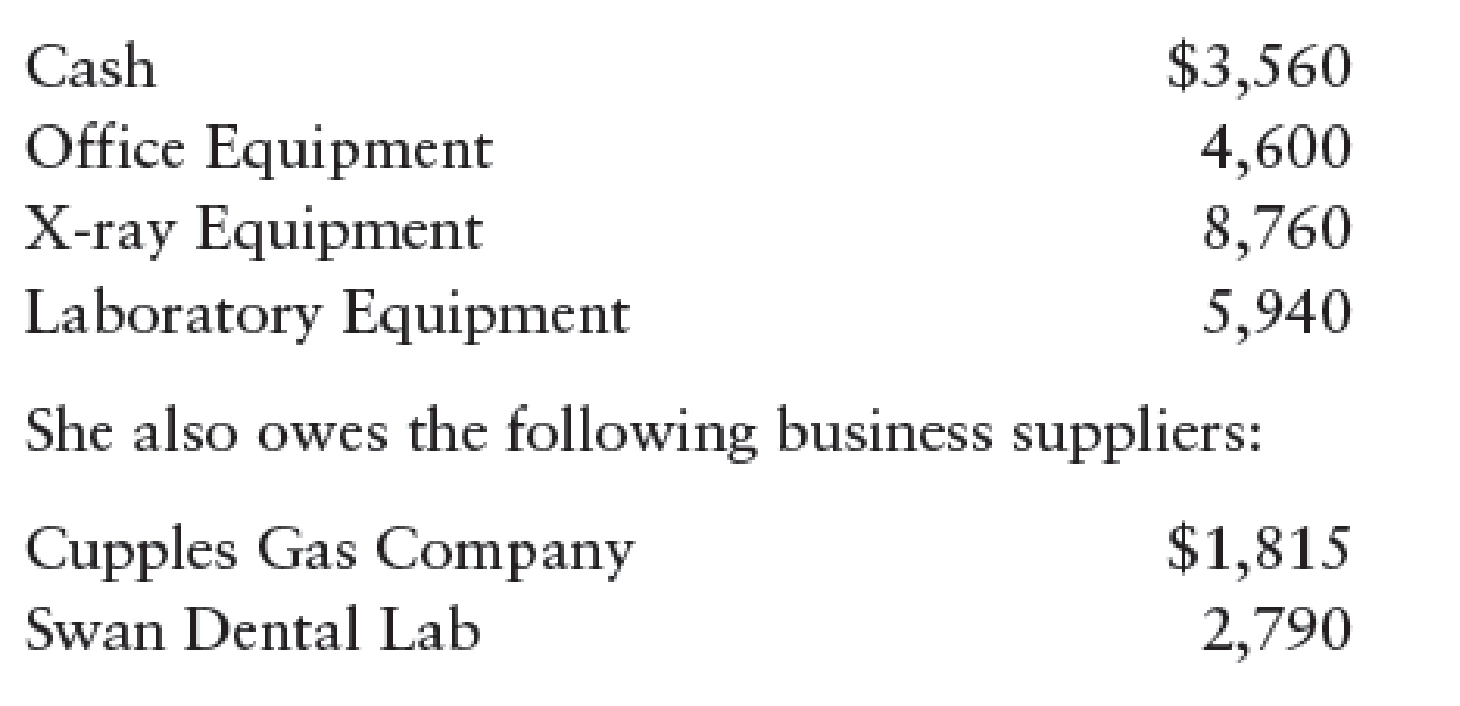Chapter 2, Problem 8SPB

Chapter
Section
Textbook Problem

SERIES B PROBLEMSTHE ACCOUNTING EQUATION Dr. Patricia Parsons is a dentist. As of January 31, Parsons owned the following property that related to her professional practice:REQUIRED1. From the preceding information, compute the accounting elements and enter them in the accounting equation as shown below.2. During February, the assets increase by $4,565, and the liabilities increase by$3,910. Compute the resulting accounting equation.3. During March, the assets decrease by $2,190, and the liabilities increase by$1,650. Compute the resulting accounting equation.

1.

To determine

Compute the accounting elements and enter them in the accounting equation.

Explanation

Accounting equation: Accounting equation is an accounting tool expressed in the form of equation, by creating a relation between resources or assets of a business and claims on the resources by the creditors, and the owners.

Figure (1)

Therefore, from the above accounting equation, it is ascertained that,

Assets($22,860)=Liabilities ($4,605)+Owners’ equity ($18,255(1)) 2. To determine Compute the resulting accounting equation if assets increase by$4,565, and the liabilities increase by $3,910. 3. To determine Compute the resulting accounting equation if assets decrease by$2,190, and the liabilities increase by \$1,650.

Still sussing out bartleby?

Check out a sample textbook solution.

See a sample solution

The Solution to Your Study Problems

Bartleby provides explanations to thousands of textbook problems written by our experts, many with advanced degrees!

Get Started

What does the invisible hand of the marketplace do?

Principles of Macroeconomics (MindTap Course List)

Should an economic model describe reality exactly?

Principles of Microeconomics (MindTap Course List)

Give some examples that illustrate how (a) seasonal factors and (b) different growth rates might distort a comp...

Fundamentals of Financial Management, Concise Edition (with Thomson ONE - Business School Edition, 1 term (6 months) Printed Access Card) (MindTap Course List)

Explain how the Kanban system helps reduce inventories.

Cornerstones of Cost Management (Cornerstones Series)